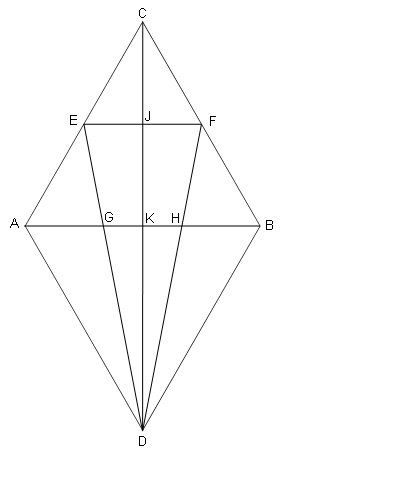### Prove: Points G and H trisect ABClick or touch to draw on the diagram
SAS (Side-Angle-Side)
The diagonals of a parallelogram bisect each other
Algebra
Definition
AC = BC = BD = AD = AB
KD = 2/3 JD
An equilateral triangle is equiangular
EF ǁ AB; EF = 1/2 AB
Side-Splitter theorem
ABCD is a rhombus
Triangles ABC, ABD equilateral; E and F midpoints of AC, BC
∠EAB = ∠FBA; ∠BAD = ∠ABD
Points G and H trisect AB
AG + BH = 2/3 AB
△AGD ≅ △BHD
Algebra
JK = 1/2 CK
All rhombuses are parallelograms
Algebra
GH = 2/3 EF
Side-Splitter theorem
Definition
The midsegment theorem
Definition
CPCTC
ASA (Angle-Side-Angle)
AG = GH = HB = 1/3 AB
Substitution
Algebra
Algebra
∠EDA = ∠FDB
CPCTC
JK = 1/2 KD
Given
ABCD is a parallelogram
AK = KB; CK = KD
GH = 1/3 AB